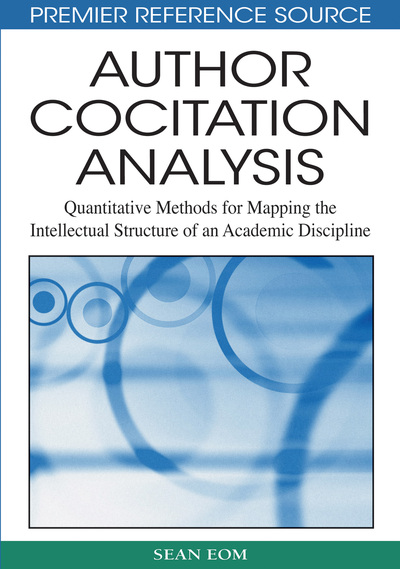# The Distance and Cluster Procedure

Sean Eom (Southeast Missouri State University, USA)
DOI: 10.4018/978-1-59904-738-6.ch009
Available
\$29.50
No Current Special Offers

## Abstract

This chapter describes the distance and cluster procedure of the SAS system. SAS version 9 introduced the proc distance procedure. All previous versions of SAS used two programs (xmacro.sas and distnew.sas) to process a transposed cocitation matrix (input) to produce a distance matrix (output). Cluster analysis is a data reduction technique for grouping various entities (individuals, variables, objects) into clusters so that the entities in the same cluster have more similarity to each other with respect to some predetermined selection criteria. The first section of this chapter explains the creation of a distance matrix, which is the input to the cluster procedure. The second part of this chapter focuses on the PROC CLUSTER statement which sets out the CLUSTER procedure steps. This chapter also includes the discussions of interpreting results of cluster analysis.
Chapter Preview
Top

## Introduction

SAS version 9 introduced the proc distance procedure. All previous versions of SAS used two programs (xmacro.sas and distnew.sas) to process a transposed cocitation matrix (input) to produce a distance matrix (output). The input to the cluster and multi-dimensional scaling analysis is a proximity matrix. The cocitation frequency counts matrix must be converted into a distance or similarity matrix. SAS version 9 created a new procedure, the distance procedure, to compute various measures of distance, dissimilarity, or similarity between the authors under investigation. The distance matrix is the input to the CLUSTER and MDS procedures.

There are many different ways of measuring inter-object similarity, including distance measures (proximity/difference between each pair of objects) and the correlation coefficient between a pair of objects. The higher cocitation frequencies between a pair of authors represent a higher level of cognitive linkages or similarities between them. In ACA, the cocitation frequency count matrix, correlation coefficient matrix, and distance matrix represent three different outputs in the same transformation process (see Table 1). Understanding input and output relations in the process helps us select the correct options in the distance and MDS procedures.

Table 1.
Summary of input/output relationships in various PROC statements
 Original input Output/input Input/output output Cocitationfrequency matrix Proc Factor Factor pattern/structure correlations Proc Distance Distance matrix Proc Cluster Clusters Proc MDS MDSConfigurationCoordinates Proc plot TwodimensionalMDS maps Proc G3D ThreedimensionalMDS maps

## Complete Chapter List

Search this Book:
Reset